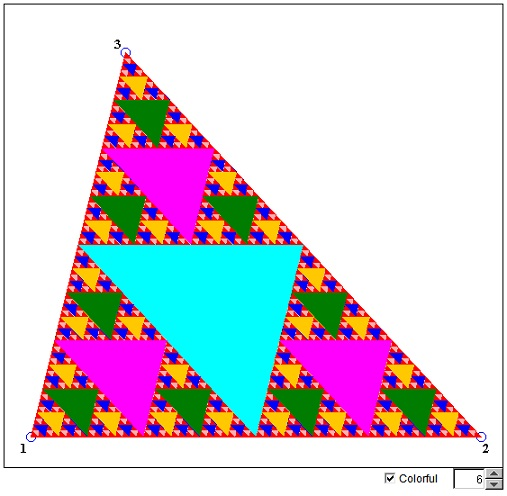# Sierpinski Gasket By Common Trema Removal

If you heard of fractals you have certainly heard of the Sierpinski triangle, or gasket. Invented by the famous Polish mathematician Waclaw Sierpinski in 1915, it became a prototypical representative of the fractals in two dimensions.

The midlines of a triangle split it into four smaller ones, equal between themselves and similar to the base trriangle. Three of them share a vertex with that triangle, whereas the middle one does not. The Sierpinski gasket is constructed iteratively and is the result of an infinite number of steps. The diagram usually drawn represent successive steps of the construction.

The basic step of the construction is the removal of the middle triangle. (The removed parts are known as tremas.) The first time it is done, three triangles remain. Next, apply the basic step to each of the remaining triangles. This leaves 9 smaller triangles to each of which we again apply the basic step to obtain 27 even smaller triangles, and so on. At the bottom of the applet below there is a control that allows you to change the number of steps, 0 through 8, and see the result.

### If you are reading this, your browser is not set to run Java applets. Try IE11 or Safari and declare the site https://www.cut-the-knot.org as trusted in the Java setup.What if applet does not run?

The curious thing about the construction is that at the end, or eventually, if you will, at the limit, the total area removed will be equal to the area of the base triangle. (The resulting series is exactly the same as in a different tremas removal procedure.) Yet, the gasket is not empty. In fact, like Cantor's set, Sierpinski's gasket contains continuum of points. One concept important to this phenomenon is measure, of which length and area are two examples. Length and area have a few similar properties: both are never negative and and both additive. If we break a stick into two pieces, the sum of their lengths will be equal to the length of the stick. If we a square is cut into two parts, their areas add up to that of the square.

The length of Sierpinski's gasket is infinite, while its area is zero. However, there exist measures that assign to the gasket a positive value. This must take into account the fractal dimension of the gasket, which is equal to log3/log2.

If we denote the base triangle T0 and subsequent sets Tk, k = 1, 2, ..., so that Tk is the union of 3k triangles each of area 4-kth of T0,we get a decreasing sequence of sets

 (1) T0T1T2...

The area of the kth term equals (3/4)k and tends to 0. However, if we consider the tremas open and the remaining triangles closed, all Tk will be compact. The family {Tk} satisfies the condition that the intersection of any finite number of the terms is not empty. (Indeed, because of (1), the intersection of the finite numbers of terms is just the term with the largest index.) As the result, the intersection of all Tk is not empty.

(Note in passing, the total perimeter of the triangles comprising Tk is (3/2)k times the perimeter of T0 and hence grows without bound.)The points in the Sierpinski gasket can be identified by their addresses. The procedure is as follows. First of all, the three triangles obtained on the basic step applied to a given triangle obtain relative addresses. I'll use symbols 1, 2, 3, but, say, letters A, B, C, or L(eft), R(ight), U(p) could be used instead. Advancing to the next step, we append the new relative address to the address obtained on the previous stage.All points of the Sierpinski gasket that belong to the left of the three triangles obtained on the first step have that addresses with "1", those that belong to the right and upper triangles have address that start with "2" and "3", respectively. Proceeding to the next step, the points in the leftmost triangle have addresses starting with "11", their neighbors on the right have addresses with "12", then triangles "21" and "22" come further right. Addresses of the points in the Sierpinski gasket that belong to the three triangles obtained from the triangle "31" on the third step, start with "311", "312", and "313", and so on.

The applet helps one grasp with this addressing mechanism. Click on black triangles will reveal their addresses. The latter serve as the common prefix for the addresses of the points in Sierpinski's gasket that lie therein.The points in the Sierpinski gasket are obtained by an infinite process. The set itself is the intersection of an infinite number of sets (each a union of an increasing number of diminishing triangles). It follows that the addresses of the points in the Sierpinski gasket are given by infinite sequences of symbols 1, 2, 3. The sequences

 (2) s1s2s3s4...

are read left to right. s1 points to one of the triangles obtained on the first step. Denote it ts1. s2 points to a subtriangle of ts1 which is denoted as ts1s2, and so on. The triangles lie within each other and shrink in size. Since there size tends to 0, there is only one point common to the whole sequence. This point is naturally represented by the address (2).### About Fractals### Related materialRead more...

• Plane Filling Curves: all possible Peano curve
• Plane Filling Curves: Hilbert's and Moore's
• Plane Filling Curves: Peano's and Wunderlich's
• Koch's Snowflake
• Cantor Sets
• Fractal Curves and Dimension
•Copyright © 1996-2018 Alexander Bogomolny

68259558